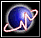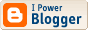Yet another weird SF fan
 I'm a mathematician, a libertarian, and a science-fiction fan. Common sense? What's that?Go to first entry

## Archives

<< current

 Yet another weird SF fan

### Another Suggestion for the American Mathematical Society

In addition to ensuring that the {subequations} environment works the way many authors think it works, it can be made more customizable by replacing the definition of {subequations} with the following:

\def\subeqform{\alph}
\def\subeqpunc{}
\newenvironment{subequations}{%
\refstepcounter{equation}%
\protected@edef\theparentequation{\theequation}%
\setcounter{parentequation}{\value{equation}}%
\setcounter{equation}{0}%
\def\theequation{\theparentequation\subeqpunc\subeqform{equation}}%
\ignorespaces
}{%
\ifnum\c@equation=0%
\setcounter{equation}{\value{parentequation}}%
\else
\setcounter{equation}{\value{parentequation}}%
\fi
\ignorespacesafterend
}

If authors want the {subequations} to be numbered (1-i), (1-ii), etc., they can simply add:
\def\subeqform{\roman}
\def\subeqpunc{-}


 Profiles My Blogger ProfileX-treme Tracker The Atom Feed